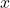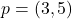# Cartesian Plane

Cartesian plane is a two dimensional co-ordinate system. This system has two axis – the x-axis and the y-axis. The center of the Cartesian plane is called the origin.

The x-axis and the y-axis divide the plane into 4 different quadrants as shown in the figure above. The axis are real number line with 0 at the origin.

Any point on the plane is plotted in terms of horizontal distance on x-axis and vertical distance on y-axis.

p = (x, y)

whereis distance on x-axis andis distance on y-axis.

Example: Plot a point for.

Solution:

\begin{aligned}&if \hspace{2px} p = (3, 5) \hspace{2px} then\\ \\
&x = 3\\ \\
&y = 5
\end{aligned}

### Pythagorean Theorem and Distance Formula

Pythagorean theorem is used for finding the distance of hypotenuse of a right triangle. The formula is modified to find the distance of two point on the Cartesian plane.

The above triangle has three sides – a, b and c, then Pythagorean theorem is given by

\begin{aligned}
&a^2 + b^2 = c^2\\ \\
&c = \sqrt{a^2 + b^2}
\end{aligned}

Suppose there are two points on the Cartesian plane.

\begin{aligned}
&p(x_1, y_1) = (2, 4)\\ \\
&q(x_2, y_2) = (2. 2)
\end{aligned}

and we have to find the distance between them.

Using Pythagorean theorem, we get

a = | y2 – y1 | = length of a

b = | x2 – x1 | = length of b

Therefore,

Distance formula for two points is

\begin{aligned}
&d = \sqrt{(|x2 - x1|)^2 +(|y2 - y1|)^2}\\ \\\
&d = \sqrt{(|2 - 2|)^2 +(|2 - 4|)^2}\\ \\
&d = \sqrt{(0)^2 + (-2)^2}\\ \\
&d = 2
\end{aligned}